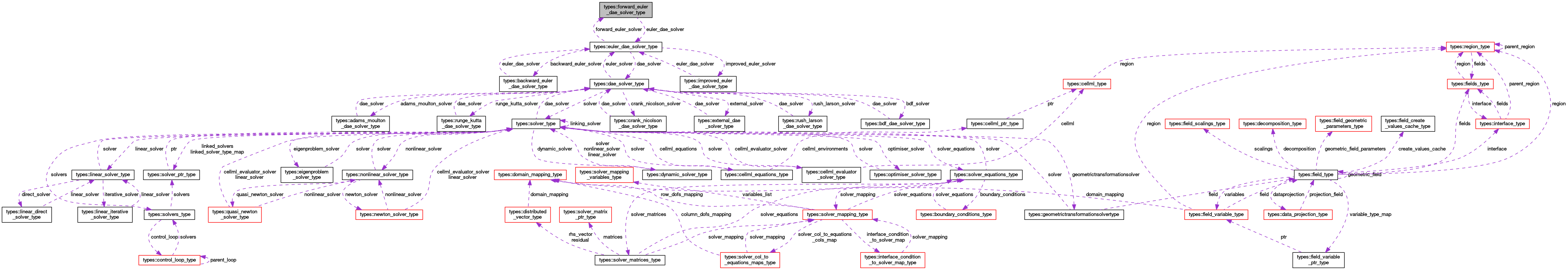OpenCMISS-Iron Internal API Documentation
types::forward_euler_dae_solver_type Type Reference

Contains information for an forward Euler differential-algebraic equation solver. More...

Collaboration diagram for types::forward_euler_dae_solver_type:[legend]

## Public Attributes

type(euler_dae_solver_type), pointer euler_dae_solver
A pointer to the differential-algebraic solver. More...

integer(intg) solver_library
The library type for the forward Euler differential-algebraic equation solver. More...

## Detailed Description

Contains information for an forward Euler differential-algebraic equation solver.

Definition at line 2510 of file types.f90.

## Member Data Documentation

 type(euler_dae_solver_type), pointer types::forward_euler_dae_solver_type::euler_dae_solver

A pointer to the differential-algebraic solver.

Definition at line 2511 of file types.f90.

 integer(intg) types::forward_euler_dae_solver_type::solver_library

The library type for the forward Euler differential-algebraic equation solver.